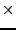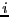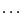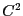Next: Storage Up: Implementation Previous: Implementation   Contents

Hessian procedure

The syntax of this function is:

Hess=INTERVAL_MATRIX IntervalHessian (int l1,int l2,INTERVAL_VECTOR & in)
This procedure should return an interval matrix of size mn, m in which the Hessian of function numbered l1 to l2 has been updated (function number start at 1). The Hessian matrix of function(which is of size nm) is stored at location Hess((-1)m+1m,1m). Remember that for each function the Hessian matrix is symmetric: this fact should be used in order to speed up the evaluation of this matrix. If a function in the system is notyou set all the elements of its hessian matrix to the interval [-1e30,1e30]. Remember also here to verify that each element of the Hessian should be interval-valuable (see section 2.1.1.3).

Jean-Pierre Merlet 2012-12-20